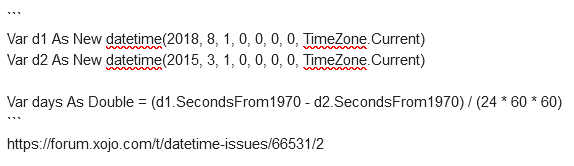# DateTime Issues

I’m trying to use the DateTime features in XoJo to determine if x days have passed since a specified date in my code. It looks like there’s a number of issues as I search. I was going to use the seconds passed since 1970, but the example code gives errors. See my two screenshots. What’s the right way to approach this to avoid what looks like some issues with XoJo and dates?

The code in the screenshot was cut and pasted from the XoJo documentation.

``````Var d1 As New datetime(2018, 8, 1, 0, 0, 0, 0, TimeZone.Current)
Var d2 As New datetime(2015, 3, 1, 0, 0, 0, 0, TimeZone.Current)

Var days As Double = (d1.SecondsFrom1970 - d2.SecondsFrom1970) / (24 * 60 * 60)
``````

You can use a DateInterval and simply subtract one DateTime object from another:

``````var d1 as DateTime = DateTime.Now
var d2 as new DateTime( 2021, 11, 6 )
var difference as DateInterval = d1 - d2
var differenceDays as Integer = difference.Days
``````

Yeah, you may need to account for cumulation using DateInterval, but here’s what your code actually gives in the DateInterval object:

Heh yeah I know, it’s not showing how many days have passed thoughSince this only accounts for days, when change the new date to next month or next year, the results don’t changeThe 5th line isn’t commented; is that a typo?
If not, it can induce the 2 following errors too. Try commenting it.

That was a typo on my part, but even with it commented out I still get the other errors.

These errors are caused by an already declare Var.
You copied two different examples from the documentation in one method…

Try

d1 = …
d2= ….

Good spot.
@Steve_Batson: you have at least two ways to figure that out:
1: a pane should have appeared at the bottom of the IDE, telling you about the error message.
2: if you move your mouse over the red crossed icon, its message should also appear.

DateInterval would be much more useful if included properties (Int64, get and set) TotalDays and TotalSeconds as most business math needs them.

2 Likes

Is it me or API 1 date were easier to use ?

Feature Request: feedback://showreport?report_id=66479

1 Like

Thanks all for the feedback. After seeing all the suggestions and comments, I finally came up with the following which meets my goal of being able to hard code a trial end date from the current date which I can set if I’m letting someone try out an app. I’d set trial end date and build just before providing to the user.

Here’s my code:

Guys, don’t paste an “image of code” when such code can be useful. Past them as text, select them, and press Control+E that they become formatted text here that can be copied/pasted after, as Julian did here, for example:

``````Var d1 As New datetime(2018, 8, 1, 0, 0, 0, 0, TimeZone.Current)
Var d2 As New datetime(2015, 3, 1, 0, 0, 0, 0, TimeZone.Current)

Var days As Double = (d1.SecondsFrom1970 - d2.SecondsFrom1970) / (24 * 60 * 60)
``````

Before rendered it looks like:Good Point.

Here’s my code as text:

``````'Get Today's Date
Var d1 As DateTime = DateTime.Now
'Set Expiration Date
Var y as integer = 2021 'Year
var m as integer = 12 'Month
var d as integer = 3 'Day
Var d2 As New DateTime(y, m, d)
' Subtract seconds since 1970 for trial end date from current date and convert to days
Var days As Double = (d2.SecondsFrom1970 - d1.SecondsFrom1970) / (24 * 60 * 60)
'If Trial is passed, show message that trial is over and quit program
dim i as integer
if days > 30 then
i=days
MessageBox("It Has Been " + i.tostring + " Days Since The Trial Period Expired!")
quit
end if
'if Trial is not over, show number of days left
If days < 30 then
i=30-days
MessageBox("Trial Period Ends In " + i.tostring + " Days")
'MessageBox("Days Left = " + Round(days).tostring)
end if
``````

Thanks for sharing! The forum has code highlighting for xojo code, you just need to select the code and press the `</>` symbol above the post editor.2 Likes
``````'Get Today’s Date
Var d1 As DateTime = DateTime.Now

'Set Expiration Date
Var y as integer = 2021 'Year
var m as integer = 12 'Month
var d as integer = 3 'Day
Var d2 As New DateTime(y, m, d)

' Subtract seconds since 1970 for trial end date from current date and convert to days
Var days As Double = (d2.SecondsFrom1970 - d1.SecondsFrom1970) / (24 * 60 * 60)

'If Trial is passed, show message that trial is over and quit program
dim i as integer
if days > 30 then
i=days
MessageBox(“It Has Been " + i.tostring + " Days Since The Trial Period Expired!”)
quit
end if

'if Trial is not over, show number of days left
If days < 30 then
i=30-days
MessageBox(“Trial Period Ends In " + i.tostring + " Days”)
'MessageBox("Days Left = " + Round(days).tostring)
end if

``````

Thanks Tim! I went ahead and edited my message even though I saw you quoted it and did it!1 Like